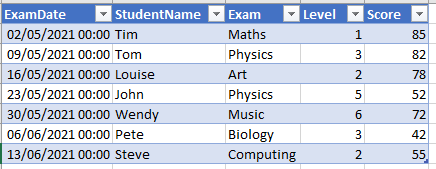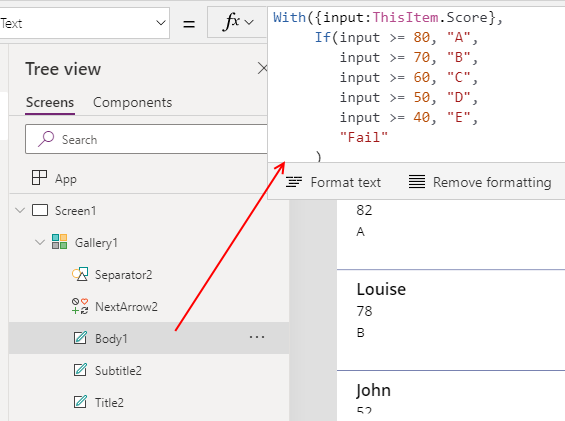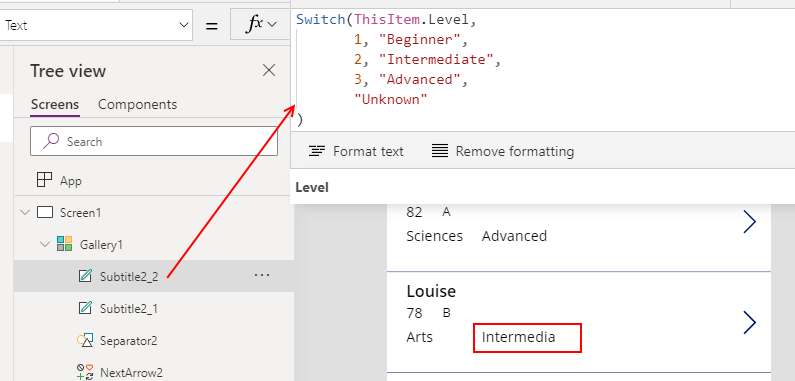# Formula - How to use the IF and Switch functions - 3 common examples

The two primary functions to carry out conditional operations are If and Switch. The typical questions that arise include - how do I match a value against numeric ranges, a list of acceptable values, or a list of matching conditions? This post highlights the example syntax that we can use.

This post summarises three frequent questions that I often see about conditional operations, and includes sample formula. In this post, we'll cover how to match a single input value against numeric bandings, how to match a single input value against a list of acceptable values, and how to match a single input value against a list of exact matches.

The If and switch statements are the two primary conditional operators in Power Apps. Confusion typically arises when app builders attempt to use the Switch function rather than If, or vice versa. For reference, the link to the online documentation is here:
For this post, we'll demonstrate the syntax using the following dataset of student exam grades.## 1. Determine where an input value belongs within a set of numeric/bounded ranges

The first common use case scenario is to determine where an input value sits within a set of ranges, or bandings. The example here would be to convert the numeric score to a grade, using the following rules:

• A - Score greater than 80
• B - Score between 60 and 70
• C - Score between 50 and 60
• D - Score between 40 and 50
• E - Score between 30 and 40
• Fail - Score < 30
The formula that carries out this task looks like this (if we were accepting the input value from a text input control called txtInputValue):

`With({input:Value(txtInputValue.Text)},     If(input >= 80, "A",        input >= 70, "B",        input >= 60, "C",        input >= 50, "D",        input >= 40, "E",        "Fail"     ))`
With this formula, we substitute 'input' with the actual input value that we want to use. As an example, the screenshot beneath illustrates how we would refer to ThisItem.Score from a gallery control.To clarify how the If function works, we pass matching sets of conditions and output values. The If function returns the first output value that matches a condition. When the function finds a matching condition, the execution of the If function terminates and Power Apps will not continue checking the remaining conditions that are specified in the call to the function.

If there are no matching conditions, the If function returns the final argument that we specify.

## 2. Determine if an input value belongs within a set of numeric/bounded ranges

The second common use case scenario is to compare an input value against a list of values. The example here would be show a category label, based the following rules:

• Sciences - Apply this label when exam name matches "Maths", "Physics", "Chemistry".
• Arts - Apply this label when exam name matches  "Music", "Art", "Dance".
• Other - Apply this label for all other exams

The formula that carries out this task looks like this. Again, we substitute 'input' with the actual input value that we want to use.
`With({input:txtInputValue.Text},     If(input in ["Maths", "Physics", "Chemistry"], "Sciences",        input in ["Music", "Art", "Dance"], "Arts",        "Other"     ))`

## 3. Testing against sets of values (existance)

In the final use case scenario, we'll examine the use of the Switch function. Unlike the examples that we've seen earlier, the Switch function is designed to match an input value against multiple conditions based on equality.

The example here would be to convert a numeric 'level' value to a label, based the following rules:

• 1 - Apply the label "Beginner"
• 2 - Apply the label "Intermediate"
• 3 - Apply the label "Advanced"
• Other values - Apply the label "Unknown"

`Switch(Value(txtInputValue.Text),       1, "Beginner",       2, "Intermediate",       3, "Advanced",       "Unknown")`

##To clarify how the Switch function works, the first value we pass defines the input value. Next, we pass pairs of values that specify the match condition and the output value. The Switch function returns the first output value where the input value is equal to the match condition. If there are no matching values, the Switch function returns the final argument that we specify.

## Conclusion

The two primary conditional operators in Power Apps are If and Switch. The Switch function is designed to compare an input value against multiple conditions based on equality. In cases where we want to compare an input against a range or list of values, the If function is more suitable.